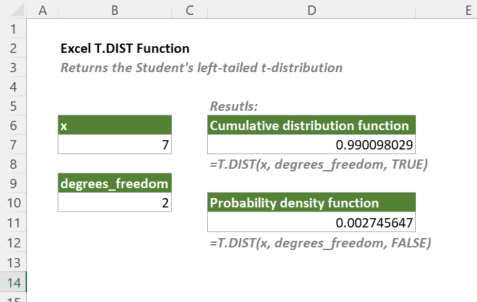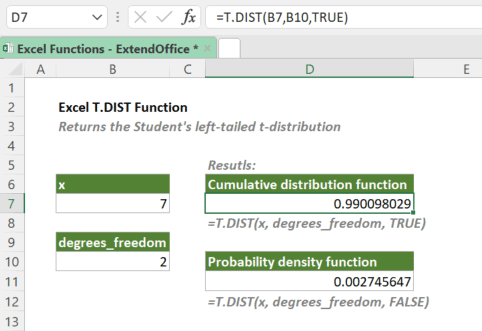## Excel T.DIST Function

The T.DIST function returns the Student's left-tailed t-distribution. The t-distribution is frequently used in testing hypotheses on small sample data sets.

Note: The T.DIST function was introduced in Excel 2010. So, it is not available in earlier versions of Excel.#### Syntax

=T.DIST(x, deg_freedom, cumulative, type)

#### Arguments

• x (required): The value at which you want to calculate the distribution.
• deg_freedom (required): An integer indicating the number of degrees of freedom. Note: deg_freedom must be ≥ 1.
• cumulative (required): A logical argument that specifies the type of distribution to be calculated:
• TRUE: Calculate the cumulative distribution function;
• FALSE: Calculate the probability density function.

#### Return Value

The T.DIST function returns a numeric value.

#### Function Notes

• If deg_freedom is not an integer, it will be truncated.
• T.DIST returns the #VALUE! error if x or deg_freedom is non-numeric.
• T.DIST returns the #DIV/0! error if cumulative is FALSE and -1 < degrees_freedom < 1.
• T.DIST returns the #NUM! error if:
• cumulative is TRUE and degrees_freedom < 1;
• cumulative is FALSE and degrees_freedom ≤ -1.

#### Example

To calculate the cumulative distribution function and probability density function for the Student's left-tailed t-distribution at 7 with 2 degrees of freedom, please copy or enter the formulas below in the corresponding result cells, and press Enter to get the results.

=T.DIST(B7,B10,TRUE)

=T.DIST(B7,B10,FALSE)Also, you can type the actual x and deg_freedom values in the formulas as shown below.

=T.DIST(7,2,TRUE)

=T.DIST(7,2,FALSE)

#### Related functions

Excel TDIST Function

Calculates the probability for Student's t-distribution with a given input (x).

Excel T.DIST.2T Function

The T.DIST.2T function returns the Student's two-tailed t-distribution. The t-distribution is frequently used in testing hypotheses on small sample data sets.

Excel T.DIST.RT Function

The T.DIST.RT function returns the Student's right-tailed t-distribution. The t-distribution is frequently used in testing hypotheses on small sample data sets.

Excel T.INV.2T Function

The T.INV.2T function returns the inverse of Student's two-tailed-distribution. The t-distribution is frequently used in testing hypotheses on small sample data sets.

Excel T.INV Function

The T.INV function returns the inverse of Student's left-tailed t-distribution. The t-distribution is frequently used in testing hypotheses on small sample data sets.

### The Best Office Productivity Tools

#### Kutools for Excel - Helps You To Stand Out From Crowd

 Popular Features: Find, Highlight or Identify Duplicates  |  Delete Blank Rows  |  Combine Columns or Cells without Losing Data  |  Round without Formula ... Super VLookup: Multiple Criteria  |  Multiple Value  |  Across Multi-Sheets  |  Fuzzy Lookup... Adv. Drop-down List: Easy Drop Down List  |  Dependent Drop Down List  |  Multi-select Drop Down List... Column Manager: Add a Specific Number of Columns  |  Move Columns  |  Toggle Visibility Status of Hidden Columns  |  Compare Columns to Select Same & Different Cells ... Featured Features: Grid Focus  |  Design View  |  Big Formula Bar  |  Workbook & Sheet Manager | Resource Library (Auto Text)  |  Date Picker  |  Combine Worksheets  |  Encrypt/Decrypt Cells  |  Send Emails by List  |  Super Filter  |  Special Filter (filter bold/italic/strikethrough...) ... Top 15 Toolsets:  12 Text Tools (Add Text, Remove Characters ...)  |  50+ Chart Types (Gantt Chart ...)  |  40+ Practical Formulas (Calculate age based on birthday ...)  |  19 Insertion Tools (Insert QR Code, Insert Picture from Path ...)  |  12 Conversion Tools (Numbers to Words, Currency Conversion ...)  |  7 Merge & Split Tools (Advanced Combine Rows, Split Excel Cells ...)  |  ... and more

Kutools for Excel Boasts Over 300 Features, Ensuring That What You Need is Just A Click Away...#### Office Tab - Enable Tabbed Reading and Editing in Microsoft Office (include Excel)

• One second to switch between dozens of open documents!
• Reduce hundreds of mouse clicks for you every day, say goodbye to mouse hand.
• Increases your productivity by 50% when viewing and editing multiple documents.
• Brings Efficient Tabs to Office (include Excel), Just Like Chrome, Edge and Firefox.